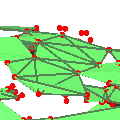CGAL 4.12 - 3D Alpha Shapes
3D Alpha Shapes ReferenceTran Kai Frank Da, Sébastien Loriot, and Mariette Yvinec
This package offers a data structure encoding either one alpha-complex or the whole family of alpha-complexes related to a given 3D Delaunay or regular triangulation. In the latter case, the data structure allows to retrieve the alpha-complex for any alpha value, the whole spectrum of critical alpha values and a filtration on the triangulation faces (this filtration is based on the first alpha value for which each face is included on the alpha-complex).

Introduced in: CGAL 2.3
Depends on: 3D Triangulations
BibTeX: cgal:dy-as3-18a
Windows Demo: 3D Alpha Shapes
Common Demo Dlls: dlls

Alpha shapes definition is based on an underlying triangulation that may be a Delaunay triangulation in case of basic alpha shapes or a regular triangulation (cf. Section 3D Regular Triangulation) in case of weighted alpha shapes.

Let us consider the basic case with a Delaunay triangulation. We first define the alpha complex of the set of points $$S$$. The alpha complex is a subcomplex of the Delaunay triangulation. For a given value of $$\alpha$$, the alpha complex includes all the simplices in the Delaunay triangulation which have an empty circumscribing sphere with squared radius equal or smaller than $$\alpha$$. Here "empty" means that the open sphere do not include any points of $$S$$. The alpha shape is then simply the domain covered by the simplices of the alpha complex (see ).

In general, an alpha complex is a non-connected and non-pure complex. This means in particular that the alpha complex may have singular faces. For $$0 \leq k \leq d-1$$, a $$k$$-simplex of the alpha complex is said to be singular if it is not a facet of a $$(k+1)$$-simplex of the complex

The alpha shapes of a set of points $$S$$ form a discrete family, even though they are defined for all real numbers $$\alpha$$. The entire family of alpha shapes can be represented through the underlying triangulation of $$S$$. In this representation, each $$k$$-simplex of the underlying triangulation is associated with an interval that specifies for which values of $$\alpha$$ the $$k$$-simplex belongs to the alpha complex. Relying on this fact, the family of alpha shapes can be computed efficiently and relatively easily. Furthermore, we can select the optimal value of $$\alpha$$ to get an alpha shape including all data points and having less than a given number of connected components.

The definition is analog in the case of weighted alpha shapes. The input set is now a set of weighted points (which can be regarded as spheres) and the underlying triangulation is the regular triangulation of this set. Two spheres, or two weighted points, with centers $$C_1, C_2$$ and radii $$r_1, r_2$$ are said to be orthogonal iff $$C_1C_2 ^2 = r_1^2 + r_2^2$$ and suborthogonal iff $$C_1C_2 ^2 < r_1^2 + r_2^2$$. For a given value of $$\alpha$$ the weighted alpha complex is formed with the simplices of the regular triangulation triangulation such that there is a sphere orthogonal to the weighted points associated with the vertices of the simplex and suborthogonal to all the other input weighted points. Once again the alpha shape is then defined as the domain covered by a the alpha complex.

CGAL provides two versions to compute alpha shapes. The first one gives access to an explicit classification of all the simplices for a fixed alpha value. The second one gives access to the entire family of alpha shapes of a set of points. This latter version comes with two modes. In the general mode, the alpha shapes correspond strictly to the definition previously made. The regularized mode provides a regularized version of the alpha shapes corresponding to the domain covered by a regularized version of the alpha complex where singular faces are removed.

## Concepts

• AlphaShapeTraits_3
• WeightedAlphaShapeTraits_3
• AlphaShapeCell_3
• AlphaShapeVertex_3
• FixedAlphaShapeTraits_3
• FixedWeightedAlphaShapeTraits_3
• FixedAlphaShapeCell_3
• FixedAlphaShapeVertex_3

## Classes

• CGAL::Alpha_status<NT>
• CGAL::Alpha_shape_3<Dt,ExactAlphaComparisonTag>
• CGAL::Alpha_shape_vertex_base_3<Traits,Vb>
• CGAL::Alpha_shape_cell_base_3<Traits,Fb>
• CGAL::Fixed_alpha_shape_3<Dt>
• CGAL::Fixed_alpha_shape_vertex_base_3<Traits,Vb>
• CGAL::Fixed_alpha_shape_cell_base_3<Traits,Fb>

Concepts

## Classes

class  CGAL::Alpha_shape_3< Dt, ExactAlphaComparisonTag >
The class Alpha_shape_3 represents the family of alpha shapes of points in the 3D space for all real $$\alpha$$. More...

class  CGAL::Alpha_shape_cell_base_3< Traits, Cb, ExactAlphaComparisonTag, WeightedTag >
The class Alpha_shape_cell_base_3 is the default model for the concept AlphaShapeCell_3. More...

class  CGAL::Alpha_status< NT >
The class Alpha_status is a small data structure to store the critical alpha values of faces of an alpha shape. More...

class  CGAL::Alpha_shape_vertex_base_3< Traits, Vb, ExactAlphaComparisonTag, WeightedTag >
The class Alpha_shape_vertex_base_3 is the default model for the concept AlphaShapeVertex_3. More...

class  CGAL::Fixed_alpha_shape_3< Dt >
The class Fixed_alpha_shape_3 represents one (fixed) alpha shape of points in the 3D space for a real $$\alpha$$. More...

class  CGAL::Fixed_alpha_shape_cell_base_3< Traits, Cb >
The class Fixed_alpha_shape_cell_base_3 is the default model for the concept FixedAlphaShapeCell_3. More...

class  CGAL::Fixed_alpha_shape_vertex_base_3< Traits, Vb >
The class Fixed_alpha_shape_vertex_base_3 is the default model for the concept FixedAlphaShapeVertex_3. More...